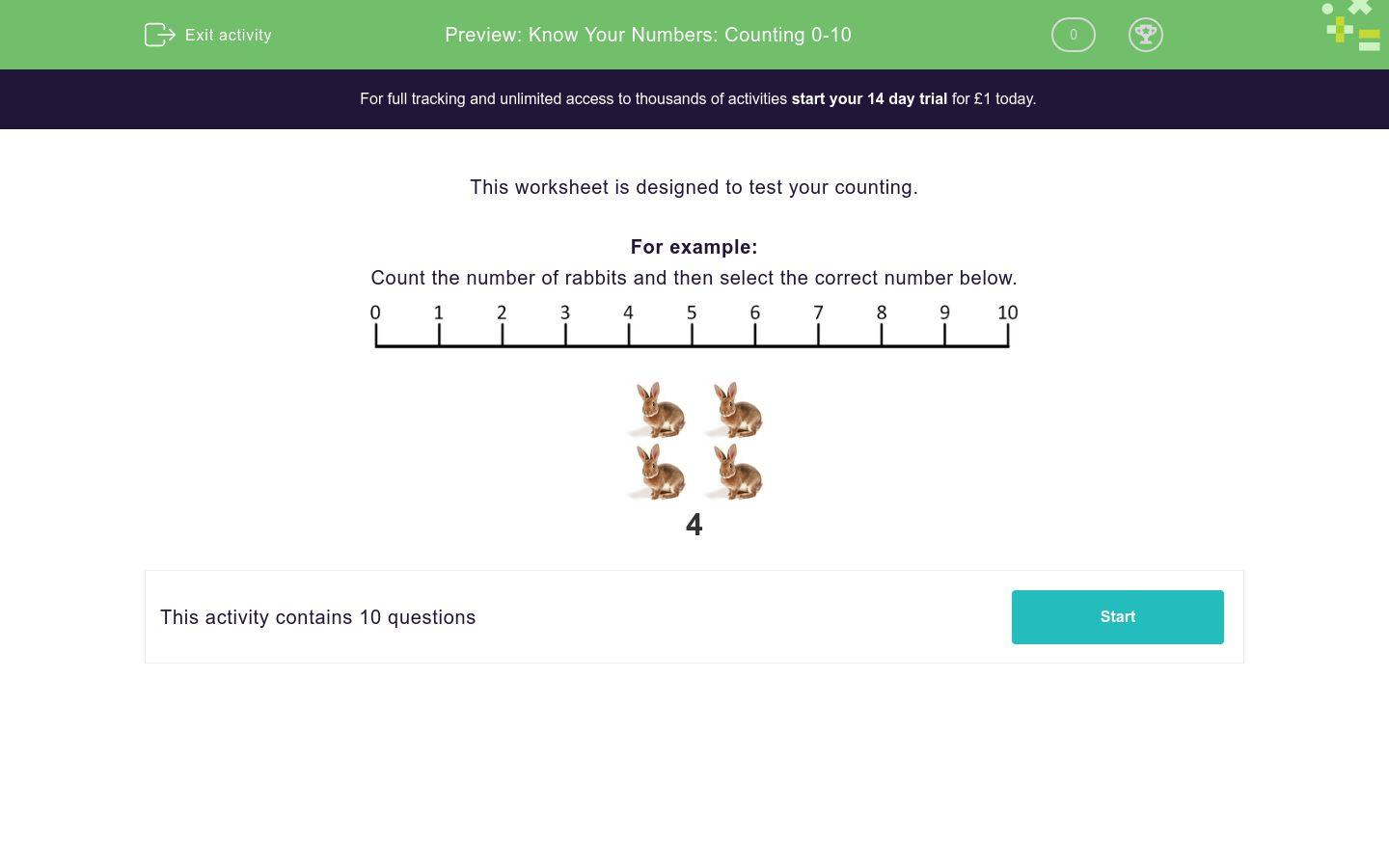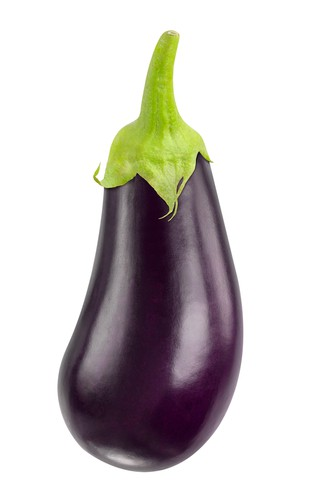# Know Your Numbers: Counting 0-10

In this worksheet, students count objects in the number range 0-10.Key stage:  KS 1

Curriculum topic:   Number: Number and Place Value

Curriculum subtopic:   Count to 100

Difficulty level:### QUESTION 1 of 10

This worksheet is designed to test your counting.

For example:

Count the number of rabbits and then select the correct number below.4

Count the number of ladies and then select the correct number below.4

2

1

3

Count the number of aubergines and then select the correct number below.3

5

2

4

Count the number of butterflies and then select the correct number below.4

2

5

3

Count the number of sheep and then select the correct number below.3

1

2

4

Count the number of cats and then select the correct number below.3

4

5

2

Count the number of apples and then select the correct number below.2

1

4

3

Count the number of peaches and then select the correct number below.3

4

1

2

Count the number of cars and then select the correct number below.2

3

4

1

Count the number of stars and then select the correct number below.5

4

3

2

Count the number of hats and then select the correct number below.1

3

2

5

• Question 1

Count the number of ladies and then select the correct number below.2
EDDIE SAYS
• Question 2

Count the number of aubergines and then select the correct number below.3
EDDIE SAYS
There are 3 aubergines.
• Question 3

Count the number of butterflies and then select the correct number below.5
EDDIE SAYS
There are 5 butterflies.
• Question 4

Count the number of sheep and then select the correct number below.1
EDDIE SAYS
There is 1 sheep.
• Question 5

Count the number of cats and then select the correct number below.5
EDDIE SAYS
There are 5 cats.
• Question 6

Count the number of apples and then select the correct number below.2
EDDIE SAYS
There are 2 apples.
• Question 7

Count the number of peaches and then select the correct number below.4
EDDIE SAYS
There are 4 peaches.
• Question 8

Count the number of cars and then select the correct number below.1
EDDIE SAYS
There is 1 car.
• Question 9

Count the number of stars and then select the correct number below.5
EDDIE SAYS
There are 5 stars.
• Question 10

Count the number of hats and then select the correct number below.2
EDDIE SAYS
There are 2 hats.
---- OR ----

Sign up for a £1 trial so you can track and measure your child's progress on this activity.

### What is EdPlace?

We're your National Curriculum aligned online education content provider helping each child succeed in English, maths and science from year 1 to GCSE. With an EdPlace account you’ll be able to track and measure progress, helping each child achieve their best. We build confidence and attainment by personalising each child’s learning at a level that suits them.

Get started